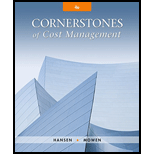# Explain why the direct materials price variance is often computed at the point of purchase rather than at the point of issuance.### Cornerstones of Cost Management (C...

4th Edition
Don R. Hansen + 1 other
Publisher: Cengage Learning
ISBN: 9781305970663

#### Solutions

Chapter
Section### Cornerstones of Cost Management (C...

4th Edition
Don R. Hansen + 1 other
Publisher: Cengage Learning
ISBN: 9781305970663
Chapter 9, Problem 7DQ
Textbook Problem
20 views

## Explain why the direct materials price variance is often computed at the point of purchase rather than at the point of issuance.

To determine

State the reason why direct materials price variance is calculated at the point of purchase rather than at the point of issuance.

### Explanation of Solution

Direct material variances: The difference between the actual material cost per unit and the standard material cost per unit for the direct material purchased is known as direct material cost variance. The direct material variance can be classified as follows:

• Direct materials price variance.
• Direct materials quantity variance.

The direct-material purchase price variance is calculated based on the quantity purchased (PQ). The deviations between the standard price and actual price are highlighted by the purchase price variance and this variance is associated to the purchasing function of the firm. In order to follow up a significant price variance a timely action is enabled by calculating this variance as soon as possible after the material is purchased...

### Still sussing out bartleby?

Check out a sample textbook solution.

See a sample solution

#### The Solution to Your Study Problems

Bartleby provides explanations to thousands of textbook problems written by our experts, many with advanced degrees!

Get Started